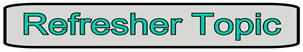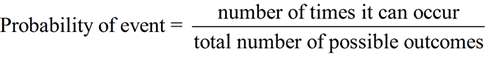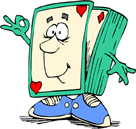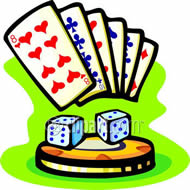Simple & Compound Events MathBitsNotebook.com Terms of Use   Contact Person: Donna RobertsDealing with ONE "outcome" ...
 Simple Event (or single event)
A simple (or single) event is an event with a single outcome (only one "answer").
 Simple Probabilities (with only one outcome (desired result)): • The probability of rolling a 3 on a die. (1/6) • The probability of drawing the ace of hearts from a deck of cards. (1/52) • The probability of tossing a head with a penny. (1/2)In a simple event, the numerator ("number of times it can occur") will be 1.Dealing with MORE THAN ONE "outcome" ...
 Compound Event
A compound event is the combination of two or more simple events (with two or more outcomes).
 Compound Probabilities (with more than one outcome (desired result) within a trial ): • The probability of rolling an even number less than 5 on a die. (2/6)     [Even numbers {2,4,6}; less than 5 {1,2,3,4}; both {2,4}] • The probability of drawing a red ace from a deck of cards. (2/52)     [red card {26 cards}; ace {4 cards}; both {red ace of hearts, red ace of diamonds}] • The probability of rolling an even number on a die, then tossing a head on a     penny. (3/12) • The probability of drawing a heart, replacing the card, then drawing a spade.     (169/2704) • The probability of tossing three pennies and getting at least 2 heads. (4/8)In a compound event, the numerator ("number of times it can occur") will be greater than 1.Remember: When dealing with probability (especially compound probability), a tree diagram will give you a visual representation of what is happening. A tree diagram will show you the solution.